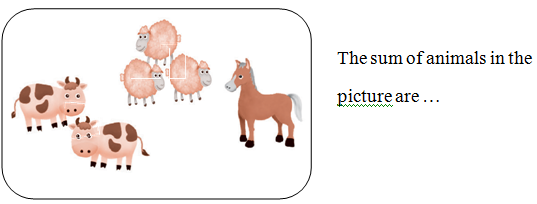Materi Kelas 1 - (Mathematics 3 September 2020)
SD Muhammadiyah 1 GKB Gresik Tahun Pelajaran 2020 - 2021
Kode Unik Siswa *
Nama Siswa *
Kelas *
RANGKUMAN MATERI
Example :
1. Write the missing numbers.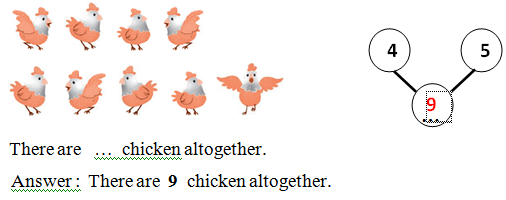2. Write the missing numbers.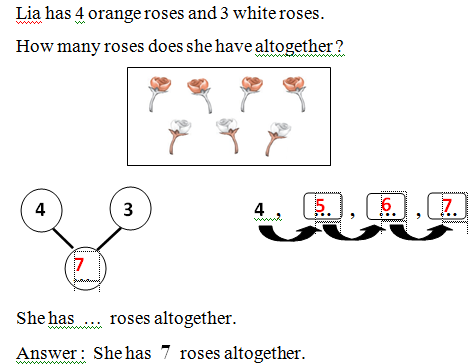Example :
1. Complete.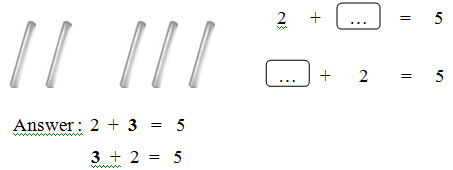2. Complete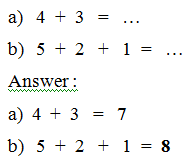Keyword
sum = jumlah
SOAL LATIHAN
1. Write the missing number. *
10 points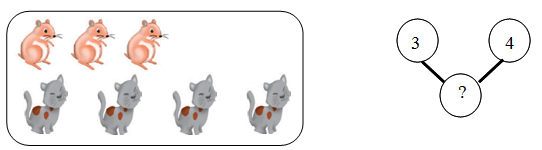2. Write the missing number. *
10 points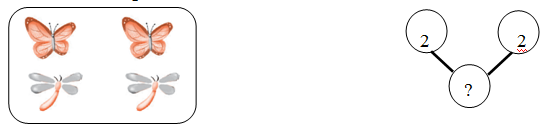3. Write the missing numbers. (count on) *
10 points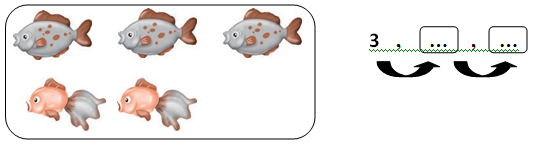4. Write the missing number. *
10 points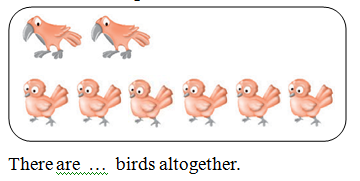5. Write the missing number. *
10 points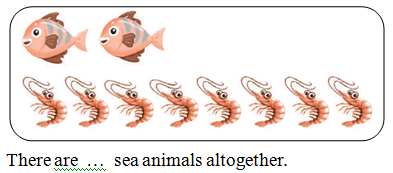6. Find the sum of 3 and 4. *
10 points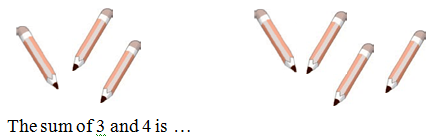7. Find the sum of 4 and 5. *
10 points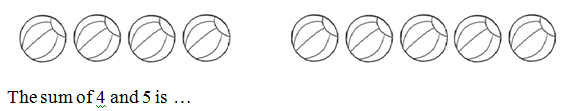8. Write the missing number. *
10 points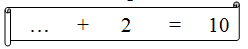9. Write the missing number. *
10 points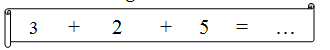10. 4 + 1 + 4 = ... *
10 points
Fill in the blank with the correct answer.
11. There are 4 boys and 1 girl *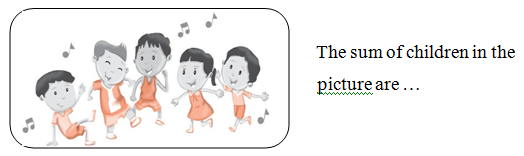12. 4rabbits are playing under tree, 2more rabbits join in. *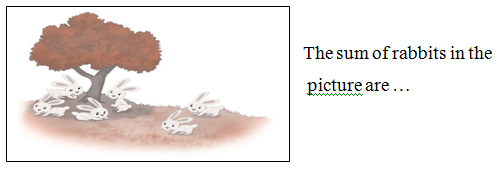13. No has 6 candies, she buys 3 more candies. *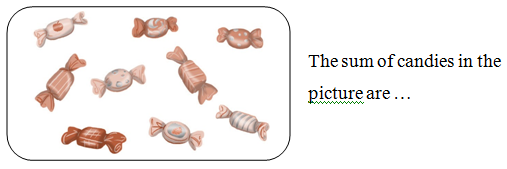14. Leon buys 2 science book, 4 mathematics books, and 2 drawing books. *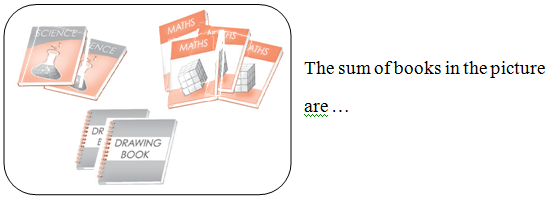15. There are 2 cows, 3 sheeps, and 1 horse. *